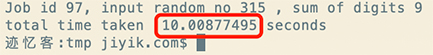迹忆客专注技术分享

Go Worker pool 工作池实现详解

Channel缓冲的重要用途之一是实现工作池。

• 创建一个 Goroutines 池，它侦听输入缓冲通道，等待分配作业
• 将作业添加到输入缓冲通道
• 作业完成后将结果写入输出缓冲通道
• 从输出缓冲通道读取和打印结果

type Job struct {
id       int
randomno int
}
type Result struct {
job         Job
sumofdigits int
}

Result 结构体有一个 job 字段，该字段是在 sumofdigits 字段中保存结果（单个数字的总和）的作业。

var jobs = make(chan Job, 10)
var results = make(chan Result, 10)

Worker Goroutines 在 jobs 缓冲通道上监听新任务。 任务完成后，将结果写入 results 缓冲通道。

func digits(number int) int {
sum := 0
no := number
for no != 0 {
digit := no % 10
sum += digit
no /= 10
}
time.Sleep(2 * time.Second)
return sum
}

func worker(wg *sync.WaitGroup) {
for job := range jobs {
output := Result{job, digits(job.randomno)}
results <- output
}
wg.Done()
}

createWorkerPool 函数将创建一个 worker Goroutines 池。

func createWorkerPool(noOfWorkers int) {
var wg sync.WaitGroup
for i := 0; i < noOfWorkers; i++ {
go worker(&wg)
}
wg.Wait()
close(results)
}

func allocate(noOfJobs int) {
for i := 0; i < noOfJobs; i++ {
randomno := rand.Intn(999)
job := Job{i, randomno}
jobs <- job
}
close(jobs)
}

func result(done chan bool) {
for result := range results {
fmt.Printf("Job id %d, input random no %d , sum of digits %d\n", result.job.id, result.job.randomno, result.sumofdigits)
}
done <- true
}

result 函数读取 results 通道并打印 Job ID，输入的随机数，以及随机数的数字总和。 result 函数还将 接收done 通道作为参数，一旦它打印了所有结果，它就会写入该通道。

func main() {
startTime := time.Now()
noOfJobs := 100
go allocate(noOfJobs)
done := make(chan bool)
go result(done)
noOfWorkers := 10
createWorkerPool(noOfWorkers)
<-done
endTime := time.Now()
diff := endTime.Sub(startTime)
fmt.Println("total time taken ", diff.Seconds(), "seconds")
}

noOfJobs 设置为 100，然后调用 allocate 将作业添加到 jobs 通道。

package main

import (
"fmt"
"math/rand"
"sync"
"time"
)

type Job struct {
id       int
randomno int
}
type Result struct {
job         Job
sumofdigits int
}

var jobs = make(chan Job, 10)
var results = make(chan Result, 10)

func digits(number int) int {
sum := 0
no := number
for no != 0 {
digit := no % 10
sum += digit
no /= 10
}
time.Sleep(2 * time.Second)
return sum
}
func worker(wg *sync.WaitGroup) {
for job := range jobs {
output := Result{job, digits(job.randomno)}
results <- output
}
wg.Done()
}
func createWorkerPool(noOfWorkers int) {
var wg sync.WaitGroup
for i := 0; i < noOfWorkers; i++ {
go worker(&wg)
}
wg.Wait()
close(results)
}
func allocate(noOfJobs int) {
for i := 0; i < noOfJobs; i++ {
randomno := rand.Intn(999)
job := Job{i, randomno}
jobs <- job
}
close(jobs)
}
func result(done chan bool) {
for result := range results {
fmt.Printf("Job id %d, input random no %d , sum of digits %d\n", result.job.id, result.job.randomno, result.sumofdigits)
}
done <- true
}
func main() {
startTime := time.Now()
noOfJobs := 100
go allocate(noOfJobs)
done := make(chan bool)
go result(done)
noOfWorkers := 10
createWorkerPool(noOfWorkers)
<-done
endTime := time.Now()
diff := endTime.Sub(startTime)
fmt.Println("total time taken ", diff.Seconds(), "seconds")
}

Job id 1, input random no 636, sum of digits 15
Job id 0, input random no 878, sum of digits 23
Job id 9, input random no 150, sum of digits 6
...
total time taken  20.025220391 seconds